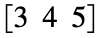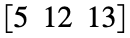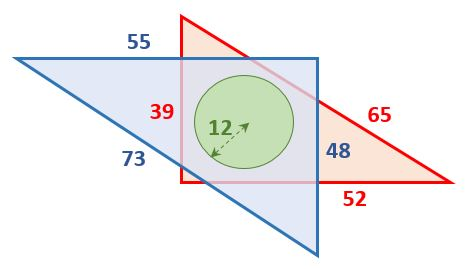# Problem 57954. Easy Sequences 108: Enclosing a Circle with Primitive Pythagorean Triangles

Pythagorean Triangle is a right triangle all sides of which are represented by integers. Examples are triangles with sidesand.
We say that pythagorean triangle is a primitive pythagorean triangle (PPT), if all of its sides are relatively prime. That is, if the sides of a pythagorean triangle are a, b and c, it is PPT if and only if: gcd(a,b) == 1 && gcd(a,c) == 1 && gcd(b,c) == 1.
Given a circle of radius R, find the dimensions of the PPT with the smallest area, that can enclose the circle, without touching the circle (i.e. no sides is a tangent of the circle, or crosses the circle). For example, in the figure below, if R = 12, the smallest pythagorean triangle that can enclose the circle without touching it is [39,52,65]. However this is not primitive since, gcd(39,52) = 13 ≠ 1. The correct smallest PPT is the triangle with dimensions: [48 55 73].-----------------------
NOTE: There can be more than one PPT with smallest area for the same value of R. For example if R = 4.5, there are two possible smallest area PPT: [20 21 29] and [12 35 37]. Both have an area of 210. In cases where there are more than one possible PPT's, the function should output the PPT with the least perimeter. So, for R = 4.5, the function output should be: [20 21 29].

### Solution Stats

100.0% Correct | 0.0% Incorrect
Last Solution submitted on Jun 06, 2023

### Community Treasure Hunt

Find the treasures in MATLAB Central and discover how the community can help you!

Start Hunting!